## Example Questions

### Example Question #7 : Right Triangles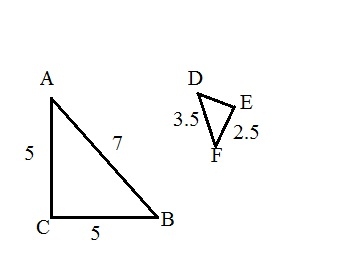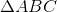is similar to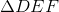. What is the measurement of segment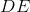?Explanation:

The word similar means, comparable in measurement, but not equal. The best sides way to compare these two triangles is by looking at the diagonal side of the triangle since it cannot be mistaken for any of the other sides of either triangle. If the larger triangle has a measurement of 7 and the smaller triangle has a measurement of 3.5 for their diagonal sides, then that means the ratio of the larger triangle to the smaller one is.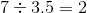This means that segment EF must be similar to segment AC (look at the orientation). So, since segment DE is similar to segment CB, divide 5 by two to get your answer.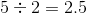### Example Question #8 : Right Triangles

There are two similar right triangles. One has side lengths of 6, 8, and 10. The other has side lengths of 24, and 18. What is the length of the missing side?Explanation:

1. Since the two triangles are similar, find the ratio of the two triangles to each other:

In this case, both triangles are multiples of a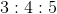special right triangle.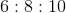and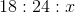The ratio of the triangles is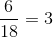2. Use the ratio you found to solve for, or the length of the missing side: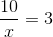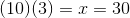### Example Question #9 : Right Triangles

There are two right triangles, Triangle A and Triangle B. Triangle A has sides with lengths of 3, 4, and 5 and Triangle B has only two given side lengths: 3 and 4. The length of the hypotenuse is not given. Are these two triangles congruent?

It is impossible to say solely based on the provided information.

No, because you do not know the angle measures.

Yes, because the missing hypotenuse length is 4.

Yes, because the missing hypotenuse length is 5.

Yes, because the missing hypotenuse length is 5.

Explanation:

1. Find the length of the missing hypotenuse:

You can do this one of two ways:

1) Using the special 3-4-5 right triangle, you can infer that the missing hypotenuse is 5.

2) By using the Pythagorean Theorem, you can solve for the length of the hypotenuse:In this case: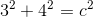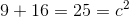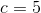2. Using the meaning of congruent (the exact same three angles and sides), determine if these two triangles fit this meaning:

They do, because both are 3-4-5 right triangles, and thus must have corresponding and equal angles due to trigonometric properties.